# NCERT Solutions for Class 9 Maths Chapter 4 Exercise 4.1 – Linear Equations in two Variables

Download NCERT Solutions for Class 9 Maths Chapter 4 Exercise 4.1 – Linear Equations in two Variables. This Exercise contains 2 questions, for which detailed answers have been provided in this note. In case you are looking at studying the remaining Exercise for Class 9 for Maths NCERT solutions for Chapter 4 or other Chapters, you can click the link at the end of this Note.

NCERT Solutions for Class 9 Maths Chapter 4 Exercise 4.1 Question 1 – Video Explanation

Class 9 Maths Chapter 4 Exercise 4.1 Question 2 – Video Explanation

### NCERT Solutions for Class 9 Maths Chapter 4 Exercise 4.1 – Linear Equations in two Variables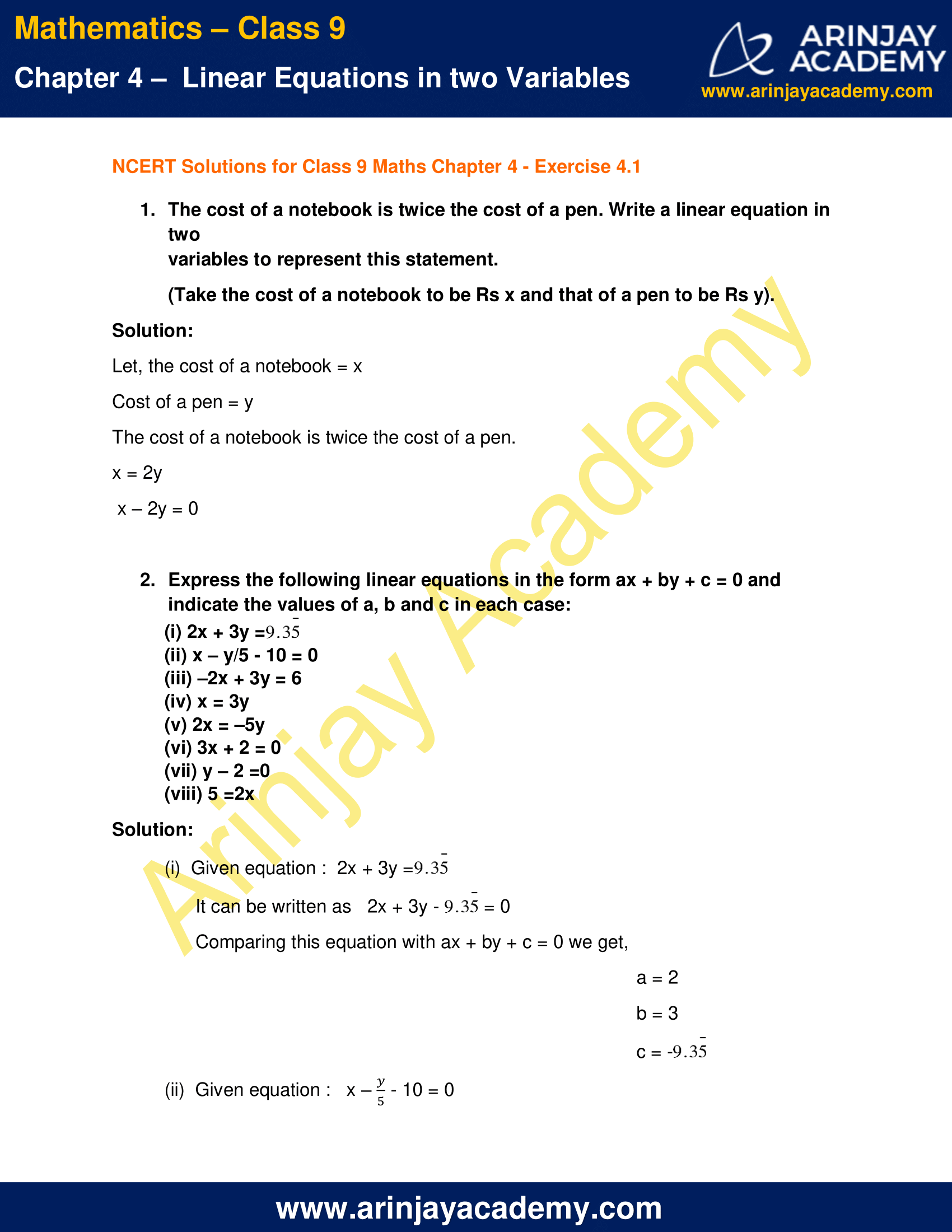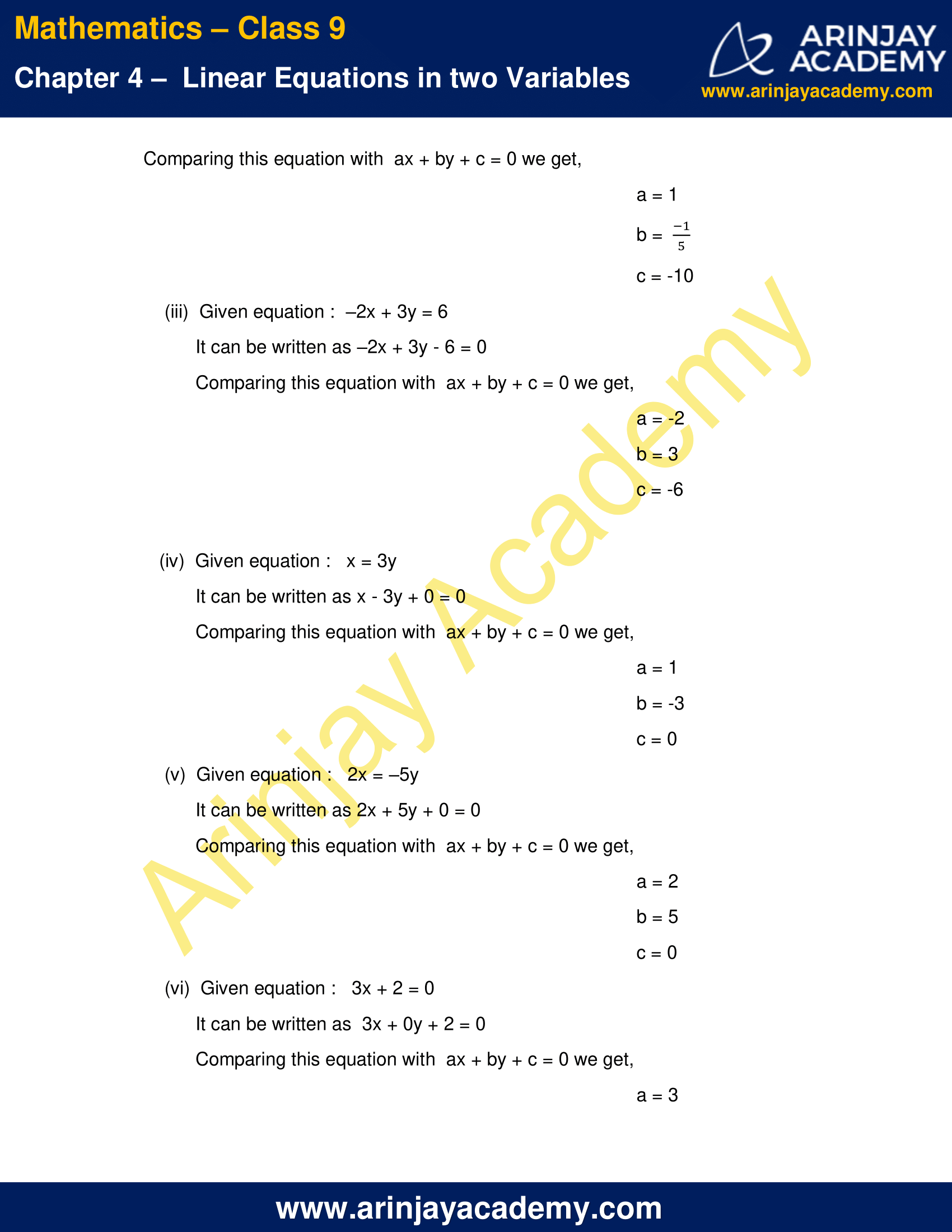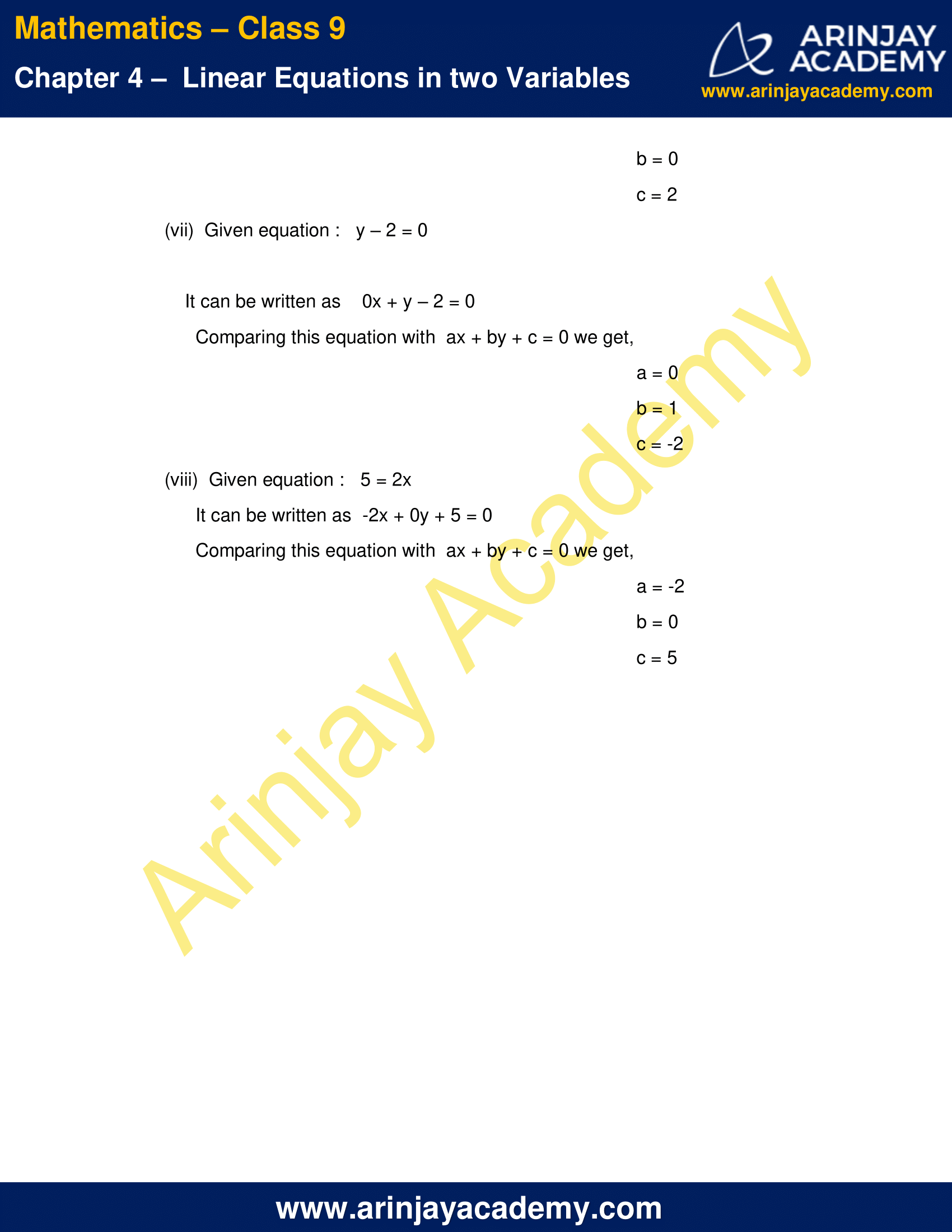NCERT Solutions for Class 9 Maths Chapter 4 Exercise 4.1 – Linear Equations in two Variables

1. The cost of a notebook is twice the cost of a pen. Write a linear equation in two variables to represent this statement.
(Take the cost of a notebook to be Rs x and that of a pen to be Rs y).

Solution:

Let, the cost of a notebook = x
Cost of a pen = y
The cost of a notebook is twice the cost of a pen.
x = 2y
x – 2y = 0

2. Express the following linear equations in the form ax + by + c = 0 and indicate the values of a, b and c in each case: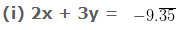(ii) x – (y/5) – 10 = 0
(iii) –2x + 3y = 6
(iv) x = 3y
(v) 2x = –5y
(vi) 3x + 2 = 0
(vii) y – 2 =0
(viii) 5 =2x

Solution: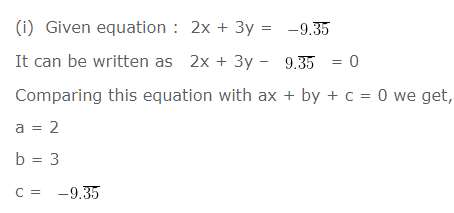(ii)  Given equation :   x – (y/5) – 10 = 0
Comparing this equation with  ax + by + c = 0 we get,
a = 1
b = (-1/5)
c = -10

(iii)  Given equation :  –2x + 3y = 6
It can be written as –2x + 3y – 6 = 0
Comparing this equation with  ax + by + c = 0 we get,
a = -2
b = 3
c = -6

(iv)  Given equation :   x = 3y
It can be written as x – 3y + 0 = 0
Comparing this equation with  ax + by + c = 0 we get,
a = 1
b = -3
c = 0

(v)  Given equation :   2x = –5y
It can be written as 2x + 5y + 0 = 0
Comparing this equation with  ax + by + c = 0 we get,
a = 2
b = 5
c = 0

(vi)  Given equation :   3x + 2 = 0
It can be written as  3x + 0y + 2 = 0
Comparing this equation with  ax + by + c = 0 we get,
a = 3
b = 0
c = 2

(vii)  Given equation :   y – 2 = 0
It can be written as    0x + y – 2 = 0
Comparing this equation with  ax + by + c = 0 we get,
a = 0
b = 1
c = -2

(viii)  Given equation :   5 = 2x
It can be written as  -2x + 0y + 5 = 0
Comparing this equation with  ax + by + c = 0 we get,
a = -2
b = 0
c = 5

NCERT Solutions for Class 9 Maths Chapter 4 Exercise 4.1 – Linear Equations in two Variables, has been designed by the NCERT to test the knowledge of the student on the topic – Linear Equations

The next Exercise for NCERT Solutions for Class 9 Maths Chapter 4 Exercise 4.2 – Linear Equations in two Variables can be accessed by clicking here.

Download NCERT Solutions for Class 9 Maths Chapter 4 Exercise 4.1 – Linear Equations in two Variables